Algebra 1 2-1 Guided Practice: Solving One-Step Equations
starstarstarstarstarstarstarstarstarstar
by Matthew Richardson
| 25 Questions1
2
1
10
Solve It! The diagram shows the amount of money that each player starts with in a video game. To be fair, each player should have the same amount of money. What amount must be in the chest? Enter only a number.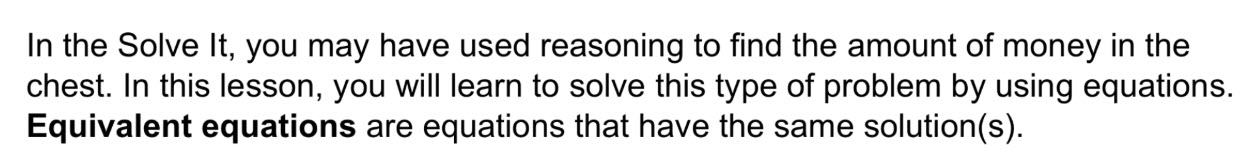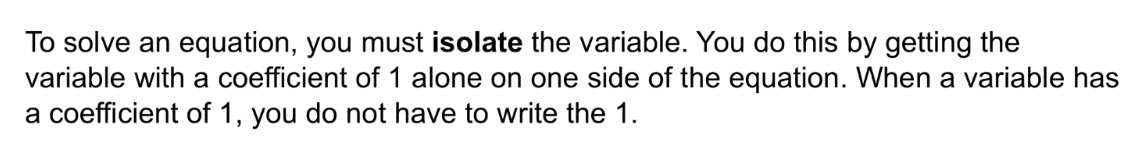3
4
5
3
2
Isolated?
Yes
No
4
2
Isolated?
Yes
No
5
2
Isolated?
Yes
No6
7
8
9
10
11
6
10
Take Note: Define equivalent equations.
7
5
Take Note: Provide an example of two equations that are equivalent equations.
8
10
Take Note: Summarize the Addition Property of Equality.
9
10
Take Note: Summarize the Subtraction Property of Equality.
10
10
Take Note: Define what it means for a variable to be isolated in an equation.
11
10
Take Note: In which of the following equations is the variable isolated? Select all that apply.
7a=21
d-5=8
-5=t

x=9
12
10
Problem 1 Got It? What is the solution of the equation? Check your answer.

-8
3
-4
-10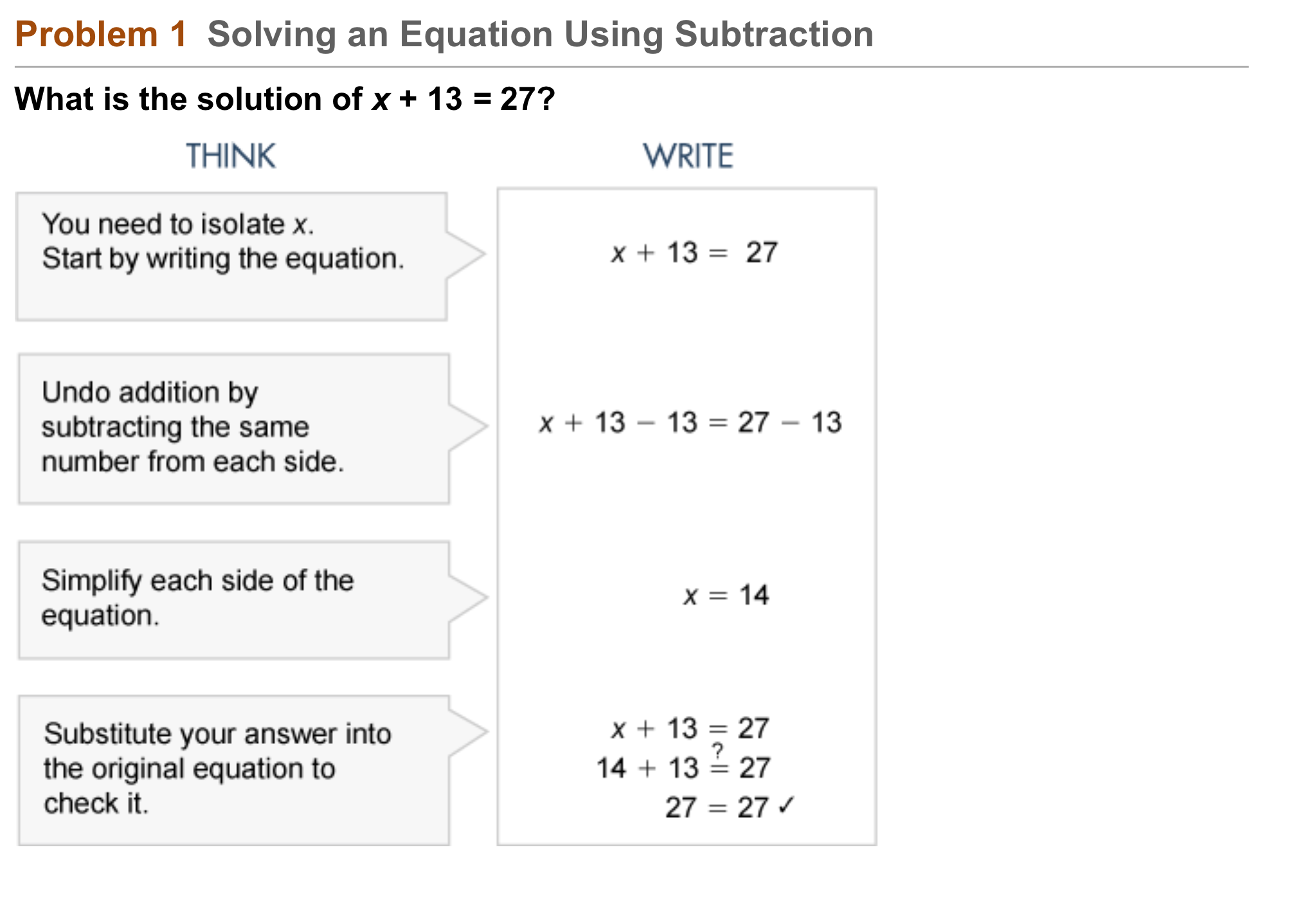1314
14
10
Problem 2 Got It?
A
B
C
D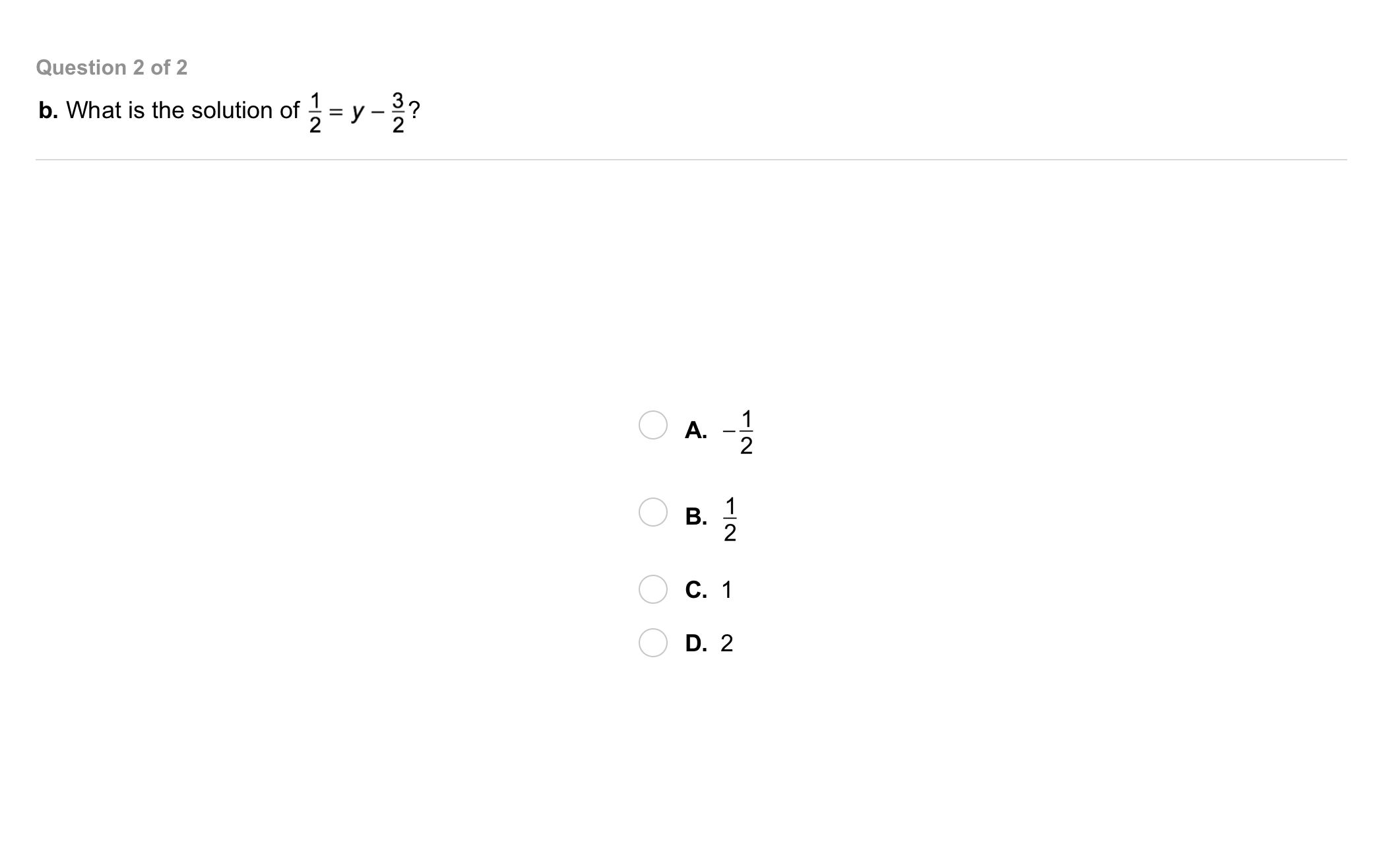15
15
10
Problem 2 Got It?
A
B
C
D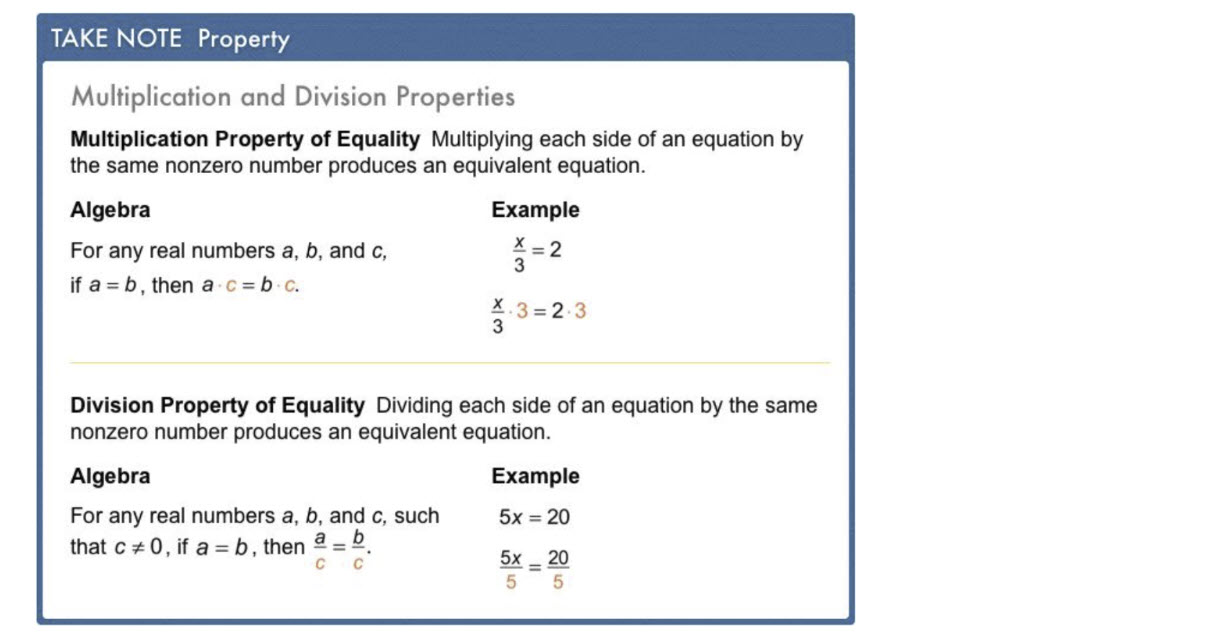16
17
16
10
Take Note: Summarize the Multiplication Property of Equality.
17
10
Take Note: Summarize the Division Property of Equality.18
18
10
Problem 3 Got It?
A
B
C
D19
19
10
Problem 3 Got It?
A
B
C
D20
20
10
Problem 4 Got It?
A
B
C
D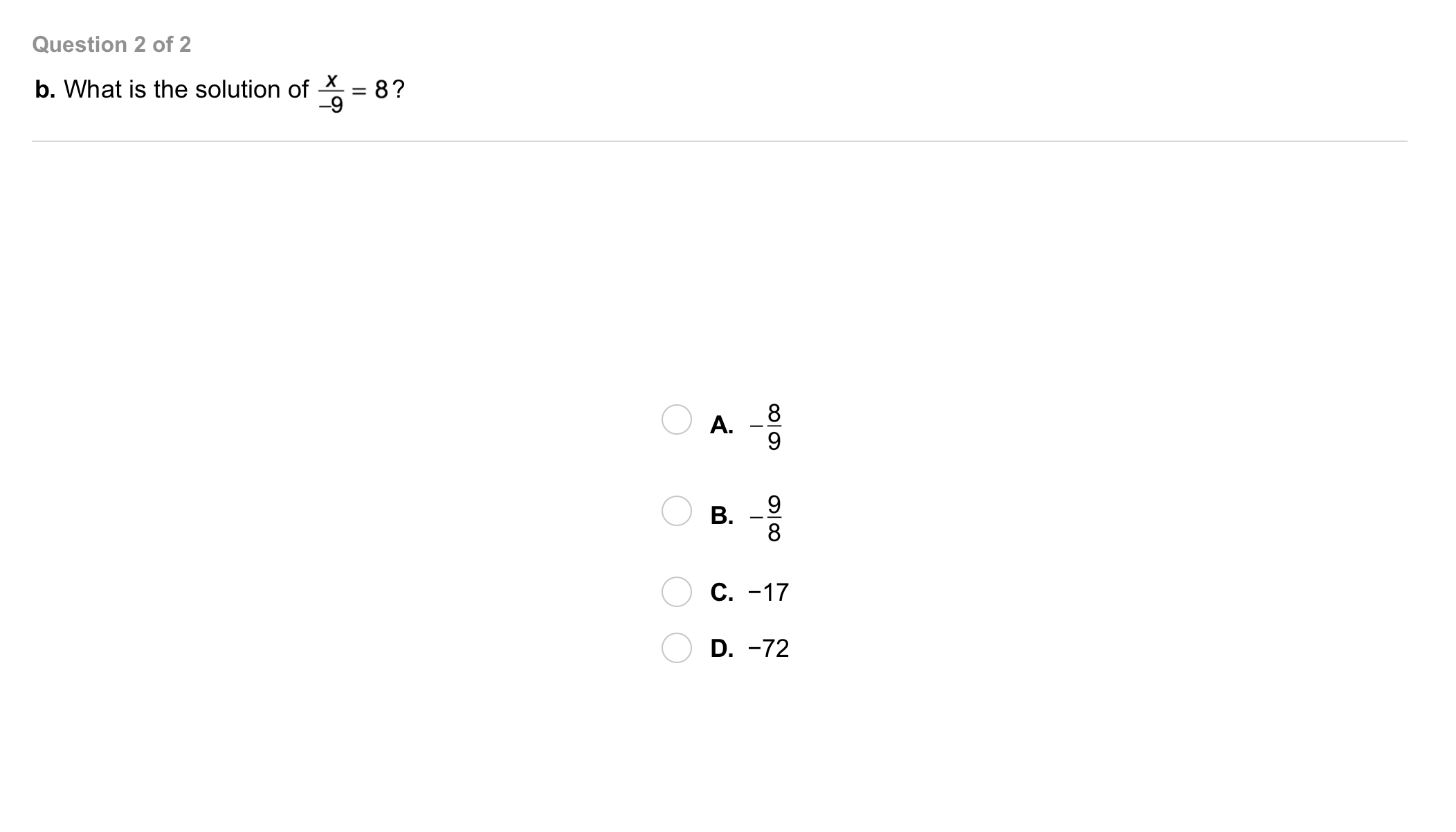21
21
10
Problem 4 Got It?
A
B
C
D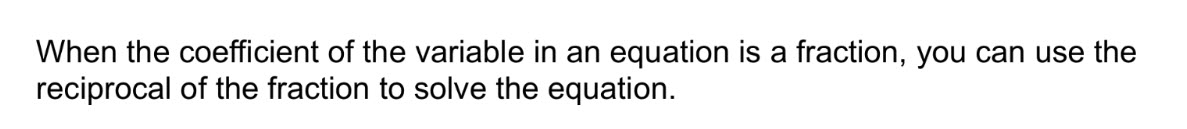22
10
Problem 5 Got It? What is the solution of the equation? Check your answer.
Enter only a number.24
24
10
Problem 6 Got It?
A
B
C
D25
25
10
Take Note: Summarize the mathematical content of this lesson. What topics, ideas, and vocabulary were introduced?